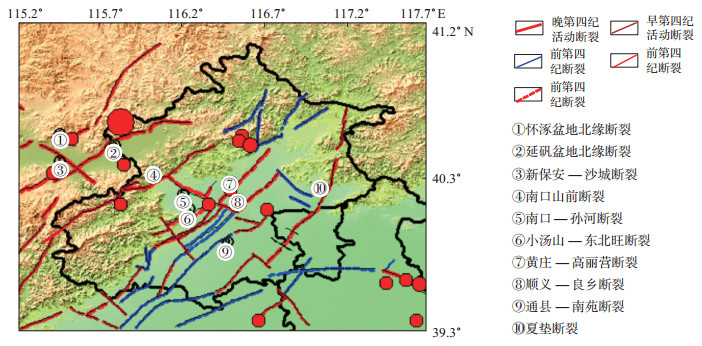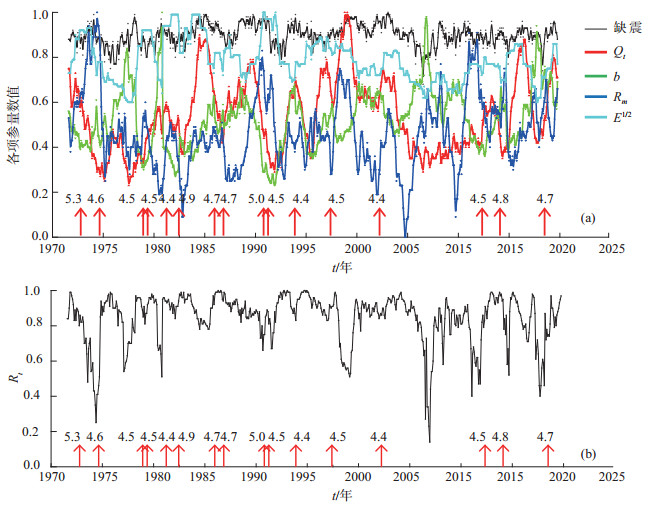﻿ 地震活动性总体参量<i>R</i><sub><i>t</i></sub>在北京及邻区地震预测中的应用文章快速检索
 地震地磁观测与研究2019, Vol. 40Issue (6): 28-34  DOI: 10.3969/j.issn.1003-3246.2019.06.0050

### 引用本文Yue Xiaoyuan, Wu Anxu, Ma Liang. Application of general parameter Rt to earthquake prediction in Beijing and its adjacent areas[J]. Seismological and Geomagnetic Observation and Research, 2019, 40(6): 28-34. DOI: 10.3969/j.issn.1003-3246.2019.06.005.### 文章历史

Application of general parameter Rt to earthquake prediction in Beijing and its adjacent areas
Yue Xiaoyuan , Wu Anxu , Ma Liang
Beijing Earthquake Agency, Beijing 100080, China
Abstract: Integrated parameter Rt is used to study the characteristics about the temporal variation of abnormal informations before moderate earthquakes in Beijing and its adjacent areas, analyzed and tracked the seismicity state of the research area before earthquakes. We discussed the characteristics about the temporal variation of precursory information before moderate earthquakes.The result shows that:when the Rt is larger than threshold 0.84, the state of seismic activity is relatively stable in the study area, the possibility of occurring moderate earthquakes is small; otherwise, when the Rt is smaller than threshold 0.84, then shows the seismic state is unstable and the possibility of occurring moderate earthquakes is more likely. The earthquake prediction efficiency of Rt value is tested, and the prediction effect is more ideal, this is significant predictor for study on seismicity state of Beijing and its adjacent areas by using integrated parameter Rt and determined the moderate risk of earthquake occurrence.
Key words: Beijing and its adjacent areas    integrated state Rt    efficiency estimation of earthquake prediction
0 引言

1 地震活动性总体状态参量Rt方法

xij为预测因子，其中i=1，2，…，5，j=1，2，…，n，表示当i为1—5时，分别表示不同的预测因子，当j为1—n时，分别表示从t1tn各个时段。为计算相关系数，每个预测因子的时间变化量用相应时间序列的最大值进行归一化处理，每个时间段Rj(t)值按下式计算。

 ${R_j} = \frac{{{S_{0j}}}}{{\sqrt {S_0^2 \cdot } \sqrt {S_j^2} }}\;\;\;\;\;\left({j = 1, \;\;2, \;\; \cdots, \;n} \right)$ (1)

 ${S_{0j}} = \frac{1}{5}\sum\limits_{i = 1}^5 {\left({{x_i} - \overline x } \right)\left({{x_{ij}} - {{\overline x }_j}} \right)}$ (2)
 $S_0^2 = \frac{1}{5}\sum\limits_{i = 1}^5 {{{\left({{x_i} - \overline x } \right)}^2}}$ (3)
 $S_j^2 = \frac{1}{5}\sum\limits_{i = 1}^5 {\left({{x_{ij}} - {{\overline x }_j}} \right)} \;\;\;\left({j = 1, \;\;2, \;\; \cdots, \;n} \right)$ (4)
 $x = \frac{1}{5}\sum\limits_{i = 1}^5 {{{\overline x }_i}}$ (5)
 ${\overline x _i} = \frac{1}{n}\sum\limits_{j = 1}^n {{x_{ij}}\;\;} \left({i = 1, \;\;2, \;\; \cdots, \;5} \right)$ (6)
 ${\overline x _j} = \frac{1}{5}\sum\limits_{i = 1}^5 {{x_{ij}}} \;\;\left({j = 1, \;\;2, \;\; \cdots, \;n} \right)$ (7)

2 研究区域及数据处理图 1 北京及邻区地形与活动断裂及ML 4.4以上地震分布 Fig.1 Distribution of topographic, active fault and ML≥4.4 earthquakes in Beijing and its adjacent areas

3 映震异常分析图 2 北京及邻区Rt值随时间变化曲线 Fig.2 Temporal variation curve of synthesized seismicity parameter Rt in Beijing and its adjacent areas

Rt≤0.84时，Rt值偏离1且波动幅度较大，北京及邻区的地震活动处于不稳定状态，相对活跃，具有发生ML 4.4以上地震的危险。据统计，自1970年以来，北京及邻区Rt值共出现12次低值异常，其中9次在异常出现后发生ML 4.4以上地震，异常演化模式为异常—恢复—发震—正常，即Rt值出现低值异常后，在异常恢复过程中发震，震后逐渐恢复至正常水平，北京及邻区地震活动恢复稳定状态，见图 2(b)表 1表 1 北京及邻区Rt低值异常与ML 4.4以上地震对应关系 Table 1 Relationship between low value anomalies of Rt and ML≥4.4 earthquakes in Beijing and its adjacent areas

4 地震预测检验

 $R = 1 - \frac{{虚报次数}}{{发出预测总次数}} - \frac{{漏报次数}}{{应预报总次数}}$ (8)

 $P = \left({n + 1} \right)/\left({N + 2} \right)$ (9)

5 结论和讨论

 边庆凯, 葛慧兰, 王娜. 2000. 缺震曲线在地震活动性分析中的应用[J]. 华北地震科学, 18(3): 45-49. DOI:10.3969/j.issn.1003-1375.2000.03.007 冀东普, 郑建常, 李冬梅, 等. 2011. 山东东部及近海地区中等以上震例前地震活动性参数群体异常研究[J]. 华北地震科学, 29(3): 28-33. DOI:10.3969/j.issn.1003-1375.2011.03.006 李永莉, 张俊伟, 刘丽芳, 等. 2004. 云南地区中强地震连发及其调制比的时空展布特征[J]. 地震研究, 27(3): 230-236. DOI:10.3969/j.issn.1000-0666.2004.03.005 陆远忠, 陈章立, 王碧泉. 1985. 地震预报的地震学方法[M]. 北京: 地震出版社, 236-267. 梅世蓉, 冯德益, 张国民. 1993. 中国地震预报概论[M]. 北京: 地震出版社. 王林瑛, 吴忠良, 陈佩燕. 2004. 地震活动性参数的相关性[J]. 地震学报, 26(Z1): 169-174. 王林瑛, 陈学忠, 朱传镇, 等. 2006. 地震活动性总体参量Rt及其在地震预测中应用的研究[J]. 地震, 26(2): 54-60. DOI:10.3969/j.issn.1000-3274.2006.02.007 王林瑛, 韩邵欣, 陈学忠. 2011. 昆仑-巴颜喀拉块体强震前后地震活动性综合参量变化特征[J]. 地震地磁观测与研究, 32(6): 1-7. DOI:10.3969/j.issn.1003-3246.2011.06.001 王炜, 宋先月, 刘铮, 等. 1999. 地震活动因子A值及其在华北地震中期预报中的运用[J]. 中国地震, 15(1): 35-45. 薛丁. 2008. 基于活动地块边界带的测震学参数Mf值对丽江地震的预测应用[J]. 地震, 28(2): 74-78. DOI:10.3969/j.issn.1000-3274.2008.02.008 张国民, 傅征祥, 桂燮泰. 2001. 地震预报概论[M]. 北京: 科学出版社.